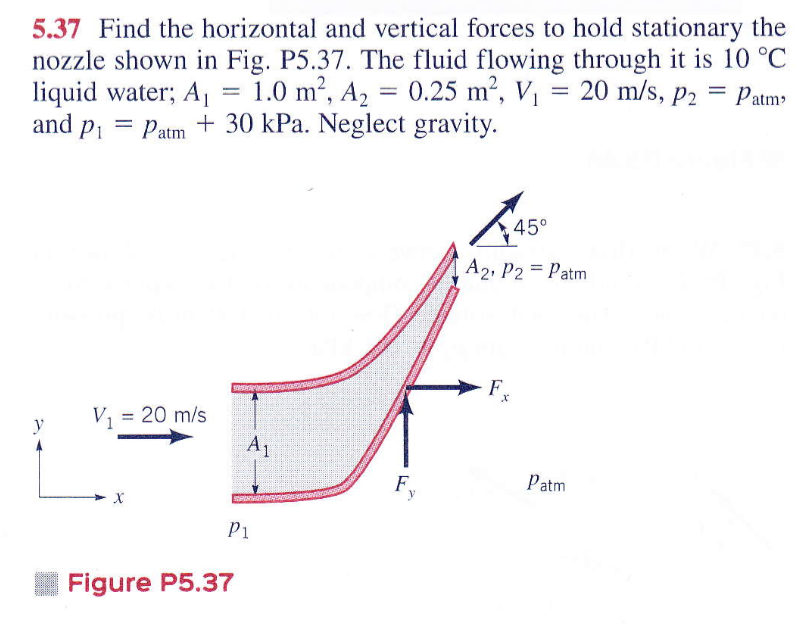# How to use the linear momentum equation to determine the forces required to hold a nozzle stationary?

This is the problem I'm trying to solve:I'm looking at the worked-out solution from my professor and it shows that the sum of the forces in the x-direction is equal to:

[d/dt(momentum) in the x-direction, out] - [d/dt(momentum) in the x-direction, in]

I don't understand what is meant by momentum out vs. momentum in and why one is positive and the other is negative.

Also, I apologize if this is a dumb question.